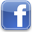Amniotic Fluid Index (AFI)

An ultrasound procedure used to asses the amount of amniotic fluid. The amniotic fluid index is measured by dividing the uterus into four imaginary quadrants (Figure 1). The linea nigra is used to divide the uterus into right and left halves.The umbilicus serves as the dividing point for the upper and lower halves. The transducer is kept parallel to the patient's longitudinal axis and perpendicular to the floor. The deepest, unobstructed, vertical pocket of fluid is measured  in each quadrant in centimeters. (Figure 2). The four pocket measurements are then added to calculate the AFI. Normal AFI values range from  5 to 25 cm [1, 4]

The amniotic fluid index (AFI) is an estimate of the amniotic fluid volume in a fetus. It is measured by adding the values of individual amniotic pocket depths (in cm's) for each of the four quadrants. It is part of the fetal biophysical profile

The normal range for amniotic fluid volumes varies with gestational age. As a rule of thumb :